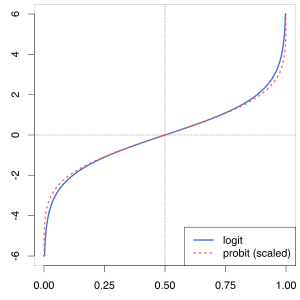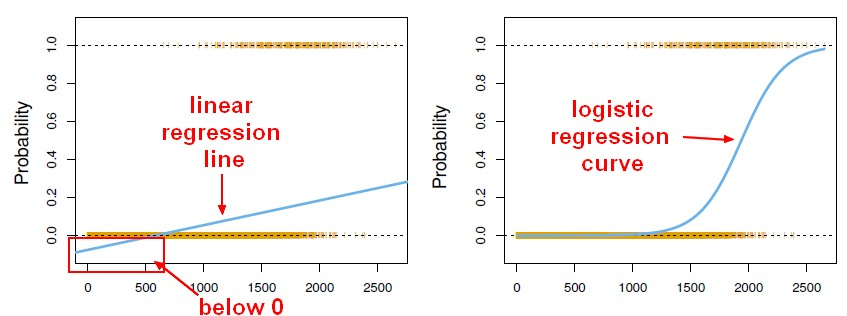# Statistics - (Logit|Logistic) (Function|Transformation)

The logit transform is a S-shaped curve that applies a softer function. It's a soft function of a step function:

• Never below 0,
• never above 1
• and a smooth transition in between.

$$\begin{array}{rrrl} Logit(x) & = & \frace^{x}}1+ e^{x}} \\ \end{array}$$

where:

• $e \approx 2:71828$ is the scientific constant, the exponential. Euler's number

The values have to lie between 0 and 1 because:

• e to anything is positive.
• As the denominator is bigger than the numerator, it's always got to be bigger than 0.
• When $x$ gets very large, this approaches 1.

Used to normalize?

The natural log of the odds is call the log-odds or logit.

## Logistic function

The logistic function (= logit ?) asymptotically approaches 0 as the input approaches negative infinity and 1 as the input approaches positive infinity. Since the results are bounded by 0 and 1, it can be directly interpreted as a probability

The logistic function $$\frac{1}{1 + \exp^{-z}}$$

Recommended PagesData Mining - Probit Regression (probability on binary problem)

Probit_modelprobit model (probability + unit) is a type of regression where the dependent variable can only take two values. As the Probit function is really similar to the logit function, the probit...Machine Learning - (Univariate|Simple) Logistic regression

A Simple Logistic regression is a Logistic regression with only one parameters. For the generalization (ie with more than one parameter), see Logistic regression comes from the fact that linear regression...Machine Learning - Logistic regression (Classification Algorithm)

The prediction from a logistic regression model can be interpreted as the probability that the label is 1. linear regression can also be used to perform classification problem. Just by transforming the...Mathematics - Sigmoid Function (S shape)

A sigmoid function is a mathematical function having an “S” shape (sigmoid curve). Often, sigmoid function refers to the logit function Sigmoid_functionProbability - Odds (Ratio)

The oddsin favor of an event is the ratio of the probability that the event will happen to the probability that the event will not happen. An odds ratio is just the probability of an event (outcome)....Statistics - Transform

fast FourierStatistics Learning - Multi-variant logistic regression

A logistic regression with multiple variables and two class outcome. Invert of the logit transformation: tilde means to be modeled as. And dot means all the other variables in the data frame...